## Example Questions

### Example Question #1 : How To Subtract Exponents

Reduce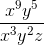to simplest form.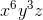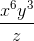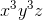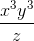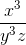Explanation:

When dividing terms with the same bases but different exponents, you will need to subtract all the pertinent exponents.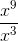becomes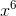,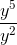becomes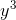,

and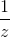stays the same because there is no other z term to combine with it.

Thus resulting in:### Example Question #1 : How To Subtract Exponents

Simplify: 32 * (423 - 421)

4^4

3^3 * 4^21

3^21

3^3 * 4^21 * 5

3^3 * 4^21 * 5

Explanation:

Begin by noting that the group (423 - 421) has a common factor, namely 421.  You can treat this like any other constant or variable and factor it out.  That would give you: 421(42 - 1). Therefore, we know that:

32 * (423 - 421) = 32 * 421(42 - 1)

Now, 42 - 1 = 16 - 1 = 15 = 5 * 3.  Replace that in the original:

32 * 421(42 - 1) = 32 * 421(3 * 5)

Combining multiples withe same base, you get:

33 * 421 * 5

### Example Question #3 : How To Subtract Exponents

Simplify. Leave no negative exponents in the final answer.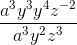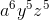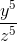None of these are the correct answer.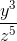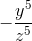Explanation:

The first step in the problem is to combine like terms in the numerator, remembering that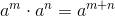: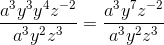Next, we resolve the numerator, using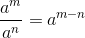and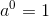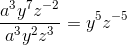Lastly, simplify the negative exponent using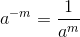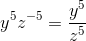Thus,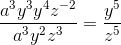### Example Question #4 : How To Subtract Exponents

Simplify to remove fractions: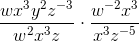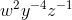None of these are correct.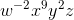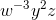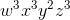Explanation:

The first step is to simplify each fraction by dividing like terms, remembering that, to get: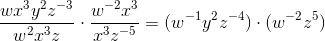Next, combine using multiplication and the rule: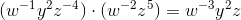Since the problem specifies that we must avoid fractions, we will not eliminate the negative exponents.

So,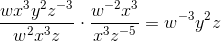### Example Question #5 : How To Subtract Exponents

Simplify the following: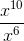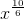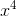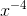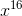Explanation:

When dividing exponential expressions with the same base, subtract the exponents. In this problem, the exponents areand. When subtracted, the result is. Thus, the correct answer is.

### Example Question #6 : How To Subtract Exponents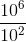can be written as which of the following?

A.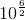B.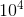C.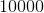A and C

A, B and C

C only

B and C

B only

B and C

Explanation:

A is not equivalent because exponents in denominators mean subtraction of exponents and not division of them. Furthermore, A, when computed, comes out to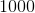instead of.

B is equivalent by the aforementioned exponential property, while C is simply computing the expression.

### Example Question #7 : How To Subtract Exponents

Simplify: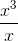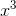In this case, subtractfrom. That yieldsas the new exponent andas the answer.